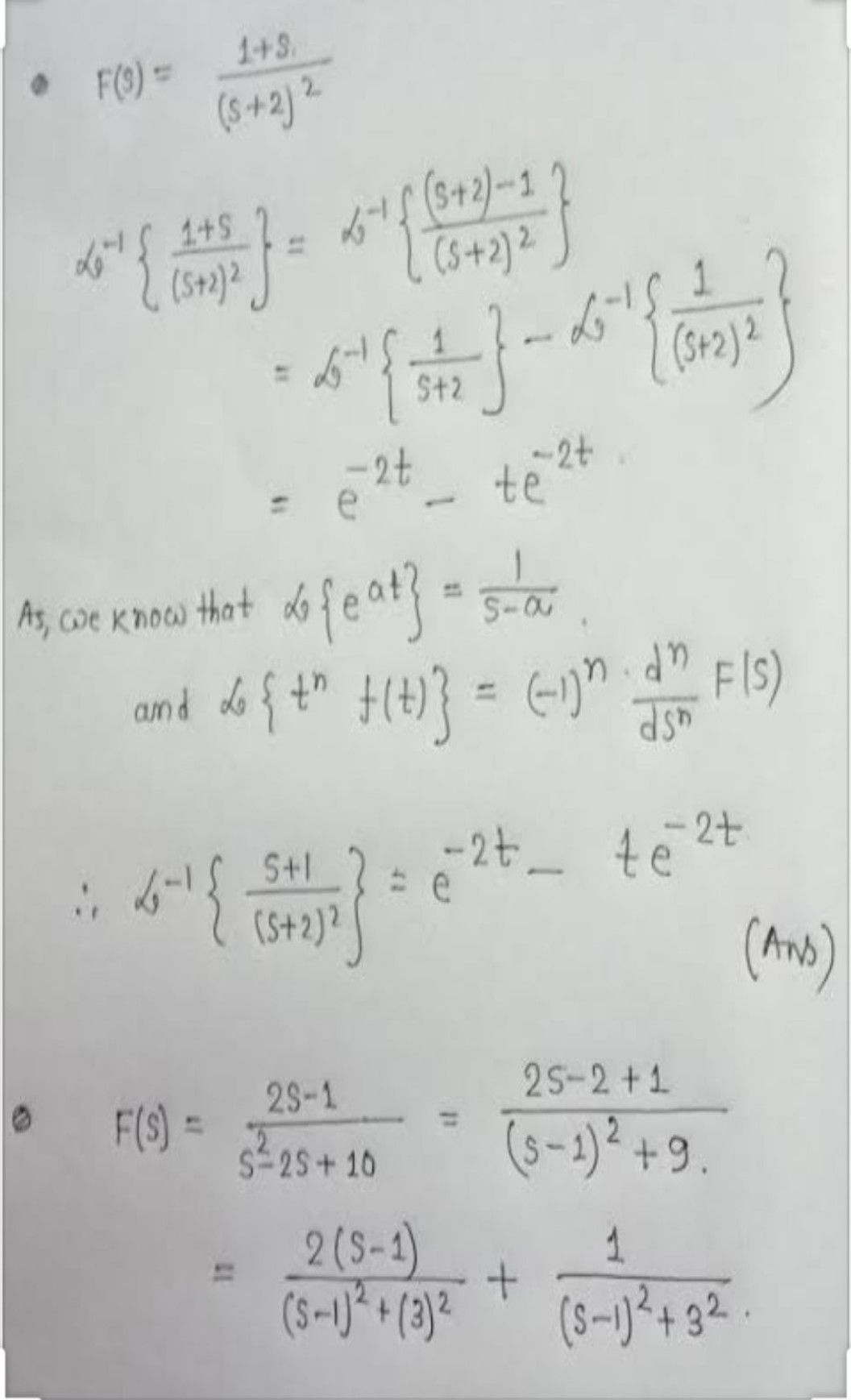Symbol
ProblemFind the inverse Loplace transform of $s+2$ $\left(5+3\right)$ $\left(s+1\right)^{3}$
Probability and Statistics
SolutionQanda teacher - nasreensk